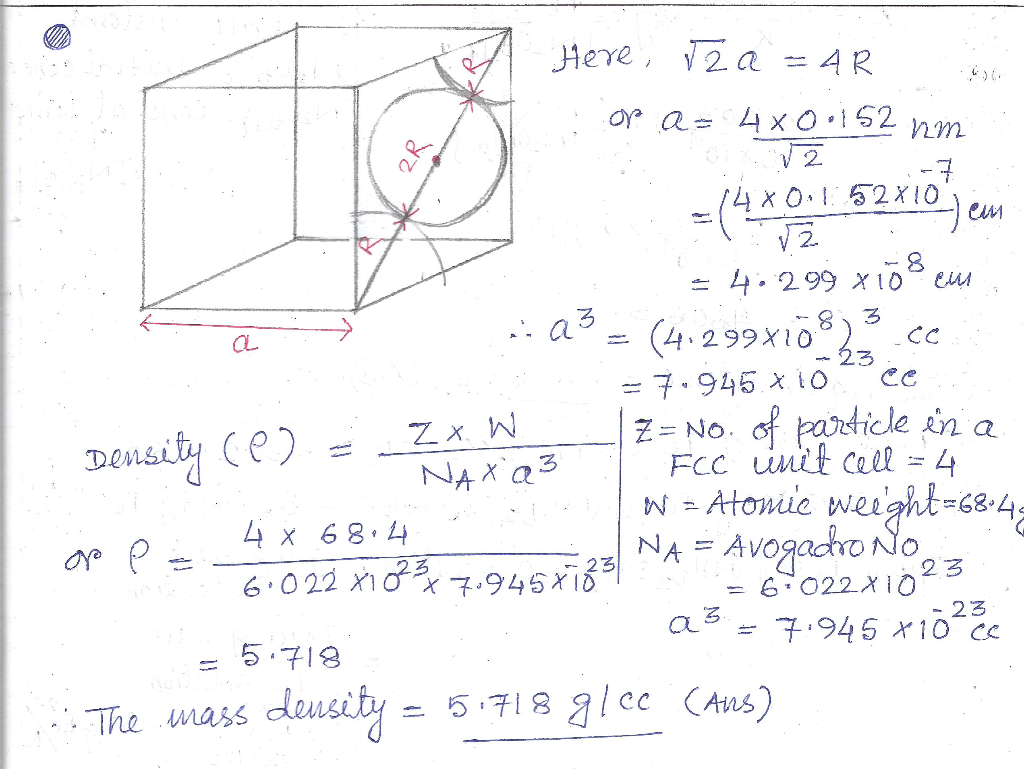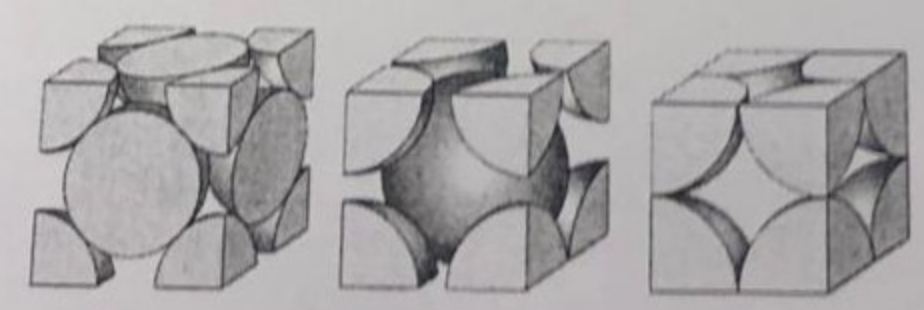Question

# Consider a face-centered cubic crystal structure that has one atom at each lattice point. The atomic...

Consider a face-centered cubic crystal structure that has one atom at each lattice point. The atomic radius, ? is 0.152 nm and the atomic weight, ? is 68.4 g/mol. Assuming the atoms to be hard spheres and touch each other with their nearest neighbor, calculate the mass density.#### Earn Coins

Coins can be redeemed for fabulous gifts.

Similar Homework Help Questions
• ### The atom radius of copper is r = 0.1278 nm. Its crystal structure has face-centered cubic...

The atom radius of copper is r = 0.1278 nm. Its crystal structure has face-centered cubic cells. a) What is the crystal-lattice constant? b) What is the concentration of copper atoms? c) What is the atomic volume (the volume of 1 mol of copper)? answer each part with explanation and shown work

• ### The crystal structure of copper is face-centered cubic (fcc), in which atoms touch along the face...

The crystal structure of copper is face-centered cubic (fcc), in which atoms touch along the face diagonal. Copper has a density of 8.92 g/cm3 . Taking Avogadro's number to be 6.022 x 1023 atoms per mole and the molar mass of copper to be 63.55 g/mol, calculate the atomic radius of a copper atom.

• ### The atomic weight per 1 mol of copper (Cu) with face-centered cubic (fcc) structure and the...

The atomic weight per 1 mol of copper (Cu) with face-centered cubic (fcc) structure and the density at 298K are 63.54 g and 8.89*10^6 g/m3, respectively. Estimate the nearest-neighbor distance of Cu atoms.

• ### A certain element exists in a face-centered cubic structure. The atomic radius of an atom of...

A certain element exists in a face-centered cubic structure. The atomic radius of an atom of this element is 121 pm. Calculate the density of this element in g/cm3. (Assume the element has a molar mass of 59.3 g/mol.)

• ### 6) A hypothetical metal has the simple cubic crystal structure. If its atomic weight is 70.6...6) A hypothetical metal has the simple cubic crystal structure. If its atomic weight is 70.6 g/mol and the atomic radius is 0.128 nm, compute its theoretical density. (N=6.022 * 1023 atoms/mol) (Theoretical density-mass of atoms in unit cell/total volume of unit cell) 7) Write down the names of each crystal structure given below.

• ### A certain element exists in a face-centered cubic structure. The atomic radius of an atom of...

A certain element exists in a face-centered cubic structure. The atomic radius of an atom of this element is 155 pm. Calculate the density of this element in g/cm3. (Assume the element has a molar mass of 55.8 g/mol.) g/cm3

• ### A certain element exists in a face-centered cubic structure. The atomic radius of an atom of...

A certain element exists in a face-centered cubic structure. The atomic radius of an atom of this element is 121 pm. Calculate the density of this element in g/cm3. (Assume the element has a molar mass of 64.2 g/mol.) g/cm3

• ### A certain element exists in a face-centered cubic structure. The atomic radius of an atom of...

A certain element exists in a face-centered cubic structure. The atomic radius of an atom of this element is 143 pm. Calculate the density of this element in g/cm3. (Assume the element has a molar mass of 62.6 g/mol.) ________ g/cm3

• ### If the atomic radius of a metal that has the face-centered cubic crystal structure is 0.137nm,...

If the atomic radius of a metal that has the face-centered cubic crystal structure is 0.137nm, calculate the volume of its unit cell.

• ### Calcium forms face centered cubic crystals. The atomic radius of a calcium atom is 197 pm....

Calcium forms face centered cubic crystals. The atomic radius of a calcium atom is 197 pm. Consider the face of a unit cell with the nuclei of the calcium atoms at the lattice points. The atoms are in contact along the diagonal. Calculate the length of an edge of this unit cell.